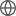Old Web
English
We construct cosmic void catalogues with the DIVE void finder upon SDSS BOSS DR12 and eBOSS DR16 galaxy samples with BAO reconstruction applied, and perform a joint BAO analysis using different types of galaxies and the corresponding voids. The BAO peak is evident for the galaxy-galaxy, galaxy-void, and void-void correlation functions of all datasets, including the ones cross correlating luminous red galaxy and emission line galaxy samples. Two multi-tracer BAO fitting schemes are then tested, one combining the galaxy and void correlation functions with a weight applied to voids, and the other using a single BAO dilation parameter for all clustering measurements of different tracers. Both methods produce consistent results with mock catalogues, and on average ~10 per cent improvements of the BAO statistical uncertainties are observed for all samples, compared to the results from galaxies alone. By combining the clustering of galaxies and voids, the uncertainties of BAO measurements from the SDSS data are reduced by 5 to 15 per cent, yielding 0.9, 0.8, 1.1, 2.3, and 2.9 per cent constraints on the distance $D_{_{\rm V}}(z)$, at effective redshifts of 0.38, 0.51, 0.70, 0.77, and 0.85, respectively. When combined with BAO measurements from SDSS MGS, QSO, and Ly$\alpha$ samples, as well as the BBN results, we obtain $H_0 = 67.58 \pm 0.91\,{\rm km}\,{\rm s}^{-1}\,{\rm Mpc}^{-1}$, $\Omega_{\rm m} = 0.290 \pm 0.015$, and $\Omega_\Lambda h^2 = 0.3241 \pm 0.0079$ in the flat-$\Lambda$CDM framework, where the 1$\,\sigma$ uncertainties are around 6, 6, and 17 per cent smaller respectively, compared to constraints from the corresponding anisotropic BAO measurements without voids and LRG-ELG cross correlations.